HOME Electronics Directory Articles/ Tutorials eBooks About Us FORUM Links Contact Us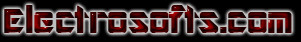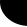### Implementing an Array of Buttons: The Shuffle Game

VC++ Programming article                       Harsha Perla and Veena Pai

### Introduction

This article came out of the result of a forum of discussion on CodeGuru.com. When we tried to implement an array of Windows buttons, we did not find any solution using ClassWizard. In VB, this can be achieved by copying a single button. Hence we discussed in the following forum: "Array of buttons in VC++."

So, we tried to have a common handler and control in the form of this game.

### To Start

• Select New->Project from the File menu. A dialog window will be displayed. Select "MFC AppWizard(exe)" and give the project name as "Shuffle".
• When you give the OK, it will ask for the type of application. Select Dialog, based on Step 1.
• Treat all other steps as default and click Finish. This will create a dialog-based application.
• Now, you see two buttons, namely "Ok", "Cancel", and a text "TODO: Place dialog controls here." Select and remove the Cancel button and the text "TODO..."
• Change the caption of the Ok button to &Exit.
• Add one more button; right-click on the button to go to Properties. Change the caption to &About and ID to IDC_About.

Now, the dialog window should look like Figure 1: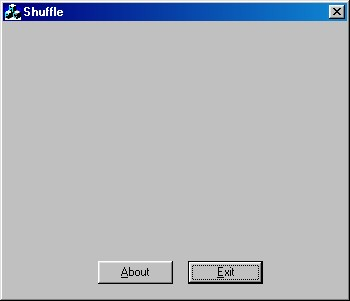Compile the project by pressing F7; then, to execute it, press Ctrl+F5. Make sure that there are no errors.

 ```void CShuffleDlg::OnAbout() { // TODO: Add your control notification handler code here //my code starts here CAboutDlg AboutDlg; AboutDlg.DoModal(); //My code ends here }```
```
```
• Now, design the window by adding 16 buttons and a Group box. Give the IDs for the buttons as IDC_But1, IDC_But2...... IDC_But16. Then, the window should look as in Figure 2.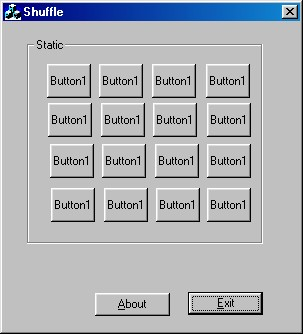• On the ClassView tab, right-click the CShuffleDlg class (if your project name is Shuffle), select to add a member variable, and give the variable type as CButton and variable name as m_b. Keep Access as public. Go to the DoDataExchange function and add the following code to it:
 ```void CShuffleDlg::DoDataExchange(CDataExchange* pDX) { CDialog::DoDataExchange(pDX); //{{AFX_DATA_MAP(CShuffleDlg) //}}AFX_DATA_MAP //my code starts here: DDX_Control(pDX, IDC_But1, m_b); DDX_Control(pDX, IDC_But2, m_b); DDX_Control(pDX, IDC_But3, m_b); DDX_Control(pDX, IDC_But4, m_b); DDX_Control(pDX, IDC_But5, m_b); DDX_Control(pDX, IDC_But6, m_b); DDX_Control(pDX, IDC_But7, m_b); DDX_Control(pDX, IDC_But8, m_b); DDX_Control(pDX, IDC_But9, m_b); DDX_Control(pDX, IDC_But10, m_b); DDX_Control(pDX, IDC_But11, m_b); DDX_Control(pDX, IDC_But12, m_b); DDX_Control(pDX, IDC_But13, m_b); DDX_Control(pDX, IDC_But14, m_b); DDX_Control(pDX, IDC_But15, m_b); DDX_Control(pDX, IDC_But16, m_b); //my code ends here } ```

This will assign the control variable for each button in the array. Now, we have created an array of 16 buttons. The thing we have to do now is to control these buttons using a program.

To play the game, we have to change the captions of the buttons to random numbers from 1 to 15. To create random numbers, we have to write a function, name it Randomize( ), and call it from OnInitDialog( ).

For that, in the ClassView tab, right-click CShuffleDlg, select add member variable; name the type as int and the variable name as m_x. Similarly, declare another variable, m_y, of the same type. Later, we use these variables to hold the position of the slider. Right-click the CShuffleDlg and select add member function; give the type as int and the function declaration as Randomize( ).

Write the following code in the function:

 ```int CShuffleDlg::Randomize() { int i=0,xx,yy; int m; CString temp; srand(time(NULL)); for(xx=0;xx<=3;xx++) for(yy=0;yy<=3;yy++) m[xx][yy]=0; while(i<16) { xx=rand()%4; yy=rand()%4; if(m[xx][yy]!=0) continue; m[xx][yy]=1; temp.Format("%d",i); m_b[xx][yy].SetWindowText(temp); if(i==0) { m_x=xx; m_y=yy; } i++; } m_b[m_x][m_y].SetButtonStyle(8); return 1; } ```

As explained above, to make the numbers randomized, we used a rand( ) function. rand( ) will generate a random integer; we made it range between 0 to 3 to select a random row and column using %4. Initial to this, we set the numbers of all the boxes to zero. Taking the numbers from 1 to 15, go on filling in the randomly selected boxes if they are not filled. Now, hide the remaining button using m_But[m_x][m_y].SetButtonStyle(8). This will set the game.

To avoid repetition of the order every time you run the program, make the seed of the random time(NULL). srand(time(NULL)) makes the random numbers dependent of time so that every time you run the program, the sequence will be different.

Now, add the following code to the OnInitDialog( ) function to randomize the number initially.

 ```BOOL CShuffleDlg::OnInitDialog() { //system generated codes // TODO: Add extra initialization here Randomize(); }```

Change the caption of the Group box to "Click On the Button to Move:".

If you compile and run, you should get the window in Figure 3: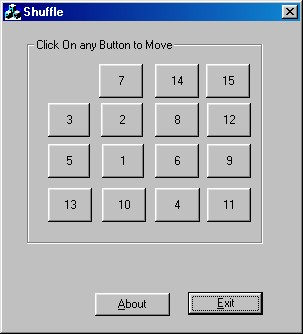Now, let us start the game. To move the button each time you click a button, you have to write a common handler for all the buttons. Go to the position where you find "BEGIN_MESSAGE_MAP(CShuffleDlg, CDialog)" (do not confuse between CAboutDlg and CShuffleDlg), and then add following code:

 ```BEGIN_MESSAGE_MAP(CShuffleDlg, CDialog) //{{AFX_MSG_MAP(CShuffleDlg) ON_WM_SYSCOMMAND() ON_WM_PAINT() ON_WM_QUERYDRAGICON() ON_BN_CLICKED(IDC_About, OnAbout) //}}AFX_MSG_MAP //my code starts here: ON_COMMAND_RANGE(IDC_But1,IDC_But16,MoveButton) //my code ends here: END_MESSAGE_MAP() ```

If we press any button, the "MoveButton" handler will be called with a parameter as the ID of the corresponding button. Now, declare the MoveButton(int btn) function of type BOOL by right-clicking the Class CShuffleDlg on the ClassView tab.

To find the row and column of the pressed button, subtract the ID of the first button from the empty button call it btn. Now, btn%4 will give the column and btn/4 will give the row.

Whenever we press a button, if the adjacent button is empty, that button should be placed on that position. For that to happen, we have to check whether the position of the empty button is adjacent. If it is, we have to change the caption of the empty button to the caption of the button pressed, show that button, and hide the button that was pressed. This will give the effect as if the desired button is moved. In m_x and m_y, store the present position of the empty button.

Add the following code to the MoveButton(int btn) function:

 ```BOOL CShuffleDlg::MoveButton(int btn) { CString no,temp; int bx,by=0; btn = btn - IDC_But1 ; by=(btn)%4; bx=(btn)/4; if(((m_x==bx) && ((m_y-by)==1)) || ((m_x==bx) && ((m_y-by)==-1)) || ((m_y==by) && ((m_x-bx)==1)) || ((m_y==by) && ((m_x-bx)==-1))) { m_b[m_x][m_y].SetButtonStyle(1,TRUE); m_b[bx][by].GetWindowText(temp); no.Format("0"); m_b[bx][by].SetWindowText(no); m_b[m_x][m_y].SetWindowText(temp); m_b[bx][by].SetButtonStyle(8,TRUE); m_x=bx; m_y=by; } return (TRUE); } ```

Now the "Shuffle Game" is ready. Play and enjoy it!